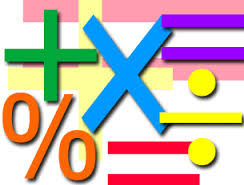# Mathematics Family

Logic Level 2If A + B means A is the mother of B; A - B means A is the brother B; A % B means A is the father of B and A x B means A is the sister of B, which of the following shows that P is the maternal uncle of Q?

×